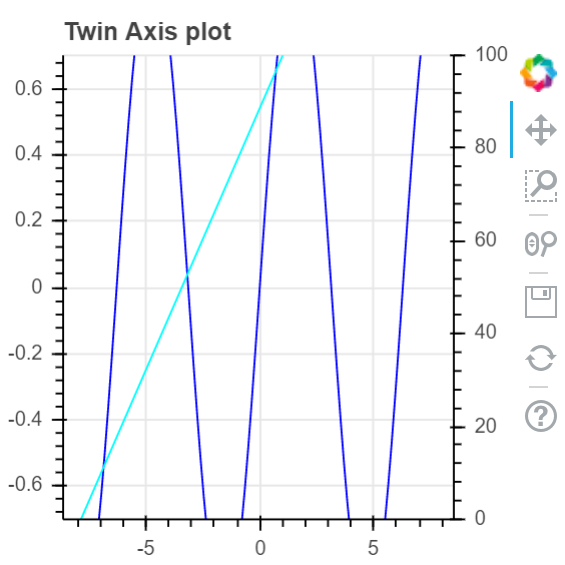# How can Bokeh library be used to visualize twin axes in Python?

PythonServer Side ProgrammingProgramming

#### Beyond Basic Programming - Intermediate Python

Most Popular

36 Lectures 3 hours

#### Practical Machine Learning using Python

Best Seller

91 Lectures 23.5 hours

#### Practical Data Science using Python

22 Lectures 6 hours

Bokeh is a Python package that helps in data visualization. It is an open source project. Bokeh renders its plot using HTML and JavaScript. This indicates that it is useful while working with web-based dashboards.

Matplotlib and Seaborn produce static plots, whereas Bokeh produces interactive plots. This means when the user interacts with these plots, they change accordingly.

Plots can be embedded as output of Flask or Django enabled web applications. Jupyter notebook can also be used to render these plots.

Installation of Bokeh on Windows command prompt

pip3 install bokeh

Installation of Bokeh on Anaconda prompt

conda install bokeh


Let us see an example −

## Example

From numpy import pi, arange, sin, linspace
x = arange(−2.5*pi, 2.5*pi, 0.15)
y = sin(x)
y2 = linspace(0, 176, len(y))
from bokeh.plotting import output_file, figure, show
from bokeh.models import LinearAxis, Range1d
my_fig = figure(title='Twin Axis plot',plot_width = 300, plot_height = 300, y_range = (−0.7, 0.7))
my_fig.line(x, y, color = "blue")
my_fig.extra_y_ranges = {"y2": Range1d(start = 0, end = 100)}
my_fig.add_layout(LinearAxis(y_range_name = "y2"), 'right')
my_fig.line(x, y2, color = "cyan", y_range_name = "y2")
show(my_fig)

## Output## Explanation

• The required packages are imported, and aliased.

• The figure function is called along with plot width and height.

• The data is generated using NumPy.

• The ‘output_file’ function is called to mention the name of the html file that will be generated.

• The ‘line’ function present in Bokeh is called, along with data.

• The ‘show’ function is used to display the plot.